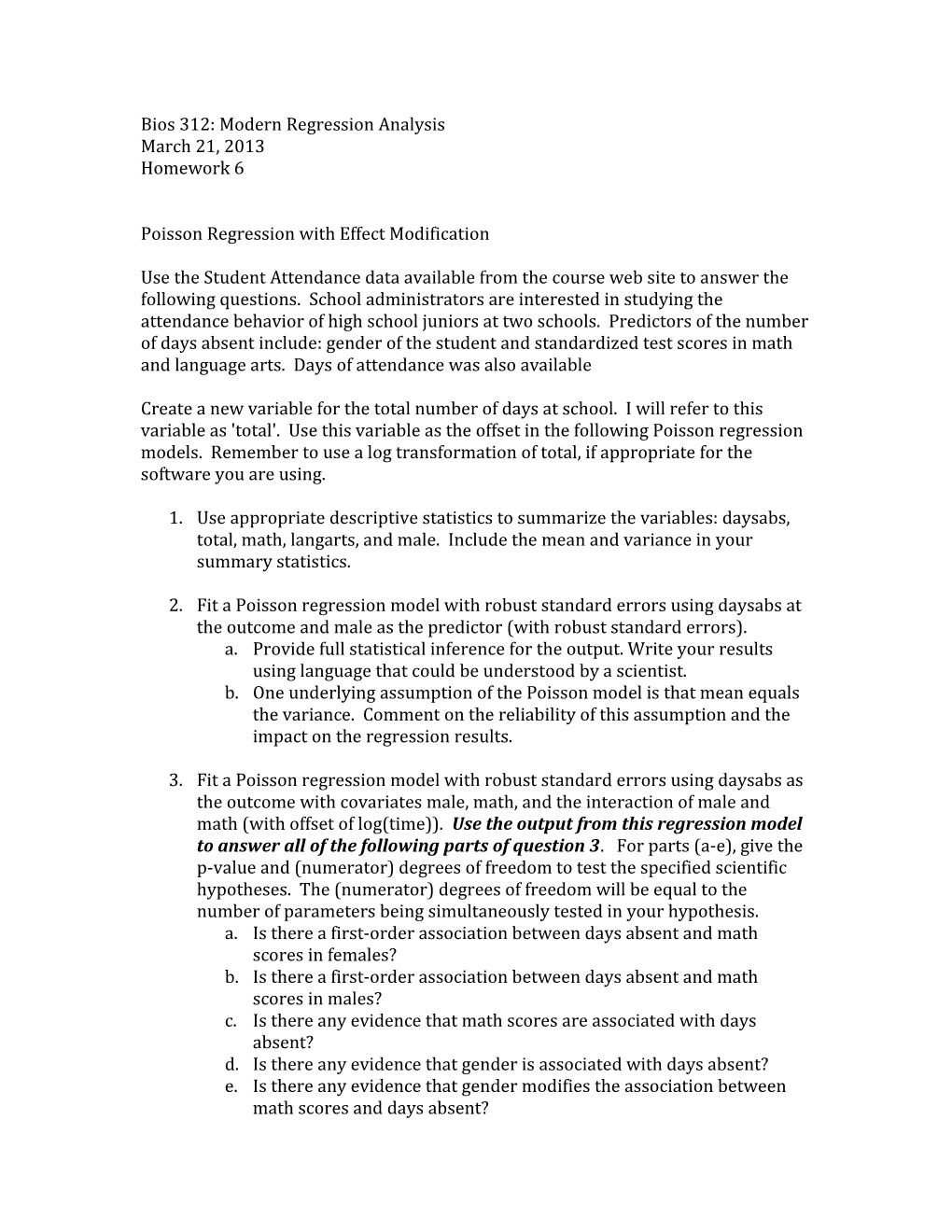# Bios 312: Modern Regression AnalysisBios 312: Modern Regression Analysis

March 21, 2013

Homework 6

Poisson Regression with Effect Modification

Use the Student Attendance data available from the course web site to answer the following questions. School administrators are interested in studying the attendance behavior of high school juniors at two schools. Predictors of the number of days absent include: gender of the student and standardized test scores in math and language arts. Days of attendance was also available

Create a new variable for the total number of days at school. I will refer to this variable as 'total'. Use this variable as the offset in the following Poisson regression models. Remember to use a log transformation of total, if appropriate for the software you are using.

1. Use appropriate descriptive statistics to summarize the variables: daysabs, total, math, langarts, and male. Include the mean and variance in your summary statistics.
1. Fit a Poisson regression model with robust standard errors using daysabs at the outcome and male as the predictor (with robust standard errors).
2. Provide full statistical inference for the output. Write your results using language that could be understood by a scientist.
3. One underlying assumption of the Poisson model is that mean equals the variance. Comment on the reliability of this assumption and the impact on the regression results.
1. Fit a Poisson regression model with robust standard errors using daysabs as the outcome with covariates male, math, and the interaction of male and math (with offset of log(time)). Use the output from this regression model to answer all of the following parts of question 3. For parts (a-e), give the p-value and (numerator) degrees of freedom to testthe specified scientific hypotheses. The (numerator) degrees of freedom will be equal to the number of parameters being simultaneously tested in your hypothesis.
2. Is there a first-order association between days absent and math scores in females?
3. Is there a first-order association between days absent and math scores in males?
4. Is there any evidence that math scores are associated with days absent?
5. Is there any evidence that gender is associated with days absent?
6. Is there any evidence that gender modifies the association between math scores and days absent?
7. What is the predicted rate of absenteeism in females with a math score of 50?
8. If the school year is 180 days long, how many days would you expect a female with a math score of 50 to miss?
9. Suppose that the data were collected only during the winter months of December and January. What assumption of the Poisson model might be false, and thus would invalidate you prediction from part g?
10. Create a plot of the predicted number of days absent in a school year (y-axis) for males and females versus possible math scores. Allow math scores to range from 0 to 100, and assume the school year is 180 days long.
1. Fit a Poisson regression model with robust standard errors using daysabs as the outcome with covariates male and langarts. Provide full statistical inference for the output. Write your answer using language that could be understood by a scientist.
1. Fit a Poisson regression model with robust standard errors using daysabs as the outcome with covariates male, math, and langarts. Provide full statistical inference for the output. Write your answer using language that could be understood by a scientist.
1. How does the scientific interpretation of male coefficient differ in models 4 and 5?